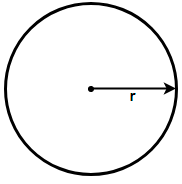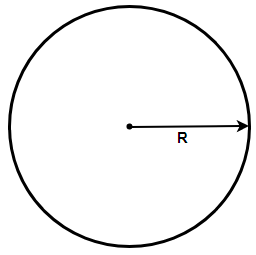Courses
Courses for Kids
Free study material
Offline Centres
MoreLast updated date: 04th Dec 2023
Total views: 281.4k
Views today: 4.81k

# What will be the increase in area of a circle, if its radius is increased by 40%?Verified
281.4k+ views
Hint: Assume the initial radius of the circle as r and the increased radius as R. To find the value of R add 40% of r with r. Now, find the increase in the numerical value of the area by subtracting the area of old circle from the area of the new circle using the relation $\left( \pi {{R}^{2}}-\pi {{r}^{2}} \right)$. Now, find the fractional increase in the area by dividing the obtained difference with the area of the old circle. Finally, multiply the obtained fraction with 100 to get the answer.

Complete step by step solution:
Here we have been asked to find the increase (percentage) in the area of a circle if we increase the radius by 40%. First we need to find the new radius of the circle.
Let us assume the radius of the initial circle is r. We know that the area of a circle is given by the formula Area = $\pi {{r}^{2}}$, so we get,$\Rightarrow$ Area of the original circle = $\pi {{r}^{2}}$
Now, let us assume the radius of the new circle as R, it is said that the radius is increased by 40% so mathematically we have the new radius given as: -
$\Rightarrow$ R = r + (40% of r)
$\Rightarrow$ R = $r+\left( \dfrac{40}{100}\times r \right)$
$\Rightarrow$ R = $\dfrac{7r}{5}$
Therefore using the formula for the area of the circle in this case we have Area = $\pi {{R}^{2}}$, so we get,$\Rightarrow$ Area of the new circle = $\pi {{\left( \dfrac{7r}{5} \right)}^{2}}$
$\Rightarrow$ Area of the new circle = $\dfrac{49\pi {{r}^{2}}}{25}$
Therefore, fractional increase in the area of the circle will be the ratio of increase in area of the circle and the area of the original circle. So we get,
$\Rightarrow$ Increase in the area = $\left( \dfrac{49\pi {{r}^{2}}}{25}-\pi {{r}^{2}} \right)$
$\Rightarrow$ Increase in the area = $\pi {{r}^{2}}\left( \dfrac{49}{25}-1 \right)$
$\Rightarrow$ fractional increase in the area = $\dfrac{\pi {{r}^{2}}\left( \dfrac{49}{25}-1 \right)}{\pi {{r}^{2}}}$
Cancelling the common factors we get,
$\Rightarrow$ fractional increase in the area = $\left( \dfrac{49}{25}-1 \right)$
$\Rightarrow$ fractional increase in the area = $\left( \dfrac{24}{25} \right)$
Therefore, multiplying the above fraction with 100 we get the percentage increase as: -
$\Rightarrow$ Percentage increase in the area = $\left( \dfrac{24}{25} \right)\times 100$
$\therefore$ Percentage increase in the area = 96
Hence, the percentage increase in the area of the circle is 96%.

Note: Always remember that the percentage increase or decrease is calculated upon the initial expression and not the final expression. This is the reason that we always take the initial expression in the denominator of the fractional increase or decrease. In case there is a decrease in the area we subtract the final area from the initial area and find the percentage decrease.